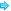科研进展新闻动态科研进展综合新闻传媒扫描
 现在位置：首页 > 新闻动态 > 科研进展
Maxwell 特征值问题的两水平预处理Helmholtz-Jacobi-Davidson方法（许学军）
2022-05-12 | 编辑：

In this paper, based on a domain decomposition (DD) method, we shall propose an efficient two-level preconditioned Helmholtz-Jacobi-Davidson (PHJD) method for solving the algebraic eigenvalue problem resulting from the edge element approximation of the Maxwell eigenvalue problem. In order to eliminate the components in orthogonal complement space of the eigenvalue, we shall solve a parallel preconditioned system and a Helmholtz projection system together in fine space. After one coarse space correction in each iteration and minimizing the Rayleigh quotient in a small dimensional Davidson space, we finally get the error reduction of this two-level PHJD method as $\gamma=c(H)(1-C\frac{\delta^{2}}{H^{2}})$, where C is a constant independent of the mesh size h and the diameter of subdomains H, $\delta$ is the overlapping size among the subdomains, and c(H) decreasing as $H\to 0$, which means the greater the number of subdomains, the better the convergence rate. Numerical results supporting our theory shall be given.

Publication:

Mathematics of Computation 91 (2022), 623-657

Author:

Qigang Liang

School of Mathematical Science, Tongji University, Shanghai 200092, People’s Republic of China

E-mail: qigang_liang@tongji.edu.cn

Xuejun Xu

Institute of Computational Mathematics, AMSS, Chinese Academy of Sciences, Beijing 100190, People’s Republic of Chinaand School of Mathematical Science, Tongji University, Shanghai  200092People’s Republic of China.

E-mail:  xxj@lsec.cc.ac.cn

【打印本页】【关闭本页】